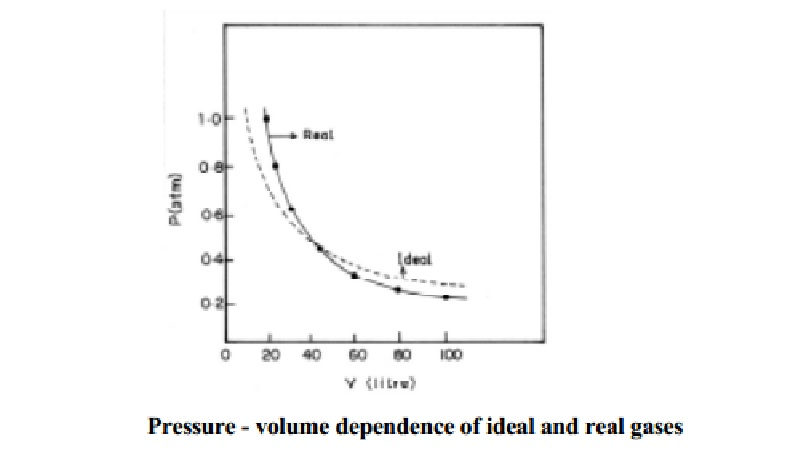Home | | Chemistry | Causes for deviation of real gas from ideal behaviour

# Causes for deviation of real gas from ideal behaviourThe perfect gas equation of state is given by PV = nRT The gases which obey this equation exactly are referred as ideal gases or perfect gases.

Causes for deviation of real gas from ideal behaviour

The perfect gas equation of state is given by

PV = nRT

The gases which obey this equation exactly are referred as ideal gases or perfect gases. Real gases do not obey the perfect gas equation exactly. Real gases show deviation because of intermolecular interaction of the gaseous molecules. Repulsive forces between the molecules cause expansion, and attractive forces cause reduction in volume. Under the conditions of low pressure and high temperature the inter-molecular interactions of the gaseous molecules are lower and tend to behave ideally under these conditions. At other conditions of pressure and volume, deviations are seen.

Volume deviation

Based on one of the postulates of the kinetic theory of the gases it is assumed that the volume occupied by the gaseous molecules themselves is negligibly small compared to the total volume of the gas. This postulate holds good for ideal gases and only under normal conditions of temperature and pressure for real gases. When temperature is lowered considerably, the total volume of the real gas decreases tremendously and becomes comparable with the actual volume of gaseous molecules. In such cases, the volume occupied by the gaseous molecules cannot be neglected in comparison with total volume of the gas.

Thus, the volume deviations created at high pressure and low temperature make the real gas to deviate from the ideal behaviour.

Pressure deviation

For an ideal gas the forces of attraction between the gaseous molecule are considered to be nil at all temperature and pressure.

For a real gas this assumption is valid only at low pressure or at high temperature. Under these conditions, the volume of the gas is high and the molecules lie far apart from one another. Therefore the intermolecular forces of attraction becomes negligible.

But at high pressure (or) at low temperature, the volume of the gas is small and molecules lie closer to one another. The intermolecular forces of attraction becomes appreciable and cannot be neglected. Therefore it is necessary to apply suitable corrections to the pressure of the real gas in the equation of state.

Study Material, Lecturing Notes, Assignment, Reference, Wiki description explanation, brief detail
11th 12th std standard Class Organic Inorganic Physical Chemistry Higher secondary school College Notes : Causes for deviation of real gas from ideal behaviour |